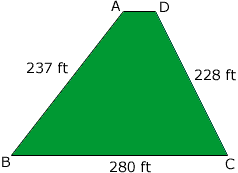Quandaries and Queries I have a peice of property that is almost a triangle but not exactly about 280 frontage with the side lines at 228 and 237   I was told I had about one acre plus a little, a developer says I have 33,000 sq ft. and I would like to know what the real result is   Jerome Hi Jerome, I can't calculate the area without more information. By "not exactly" I assume your loot looks like the diagram below.If AD and BC are parallel and you tell me the length of AD I can calculate the area. Id AD and BC are not parallel I need the length of AD but also some more information, either on of the angles or the distance from A to C or the distance from B to D. If A and D were the same point so that you have a triangle then I can use Heron's Formula to calculate the area. In this situation I got 25,974 square feet. Penny Jerome wrote back: I am sorry I gave the wrong dimensions   the front is 289    left side 237     right side 279     property is in the shape of a triangle were the left and right meet in the same place in the rear, the front is only slightly curved and is 289 Hi again Jerome, I applied Heron's Formula to the new dimensions and got an area of 30,557 square feet. An acre is 43,560 square feet and 30,557/43,560 is approximately 0.7, so the area of your lot is about seven tenths of an acre. Penny Go to Math Central